Start typing, then use the up and down arrows to select an option from the list.# Atomic Mass

Jules Bruno
198views
now we can look up. The atomic mass is off a known element by simply looking at the periodic table. But there's going to be instances where you may not know the identity of the element, or you may not have a periodic table handy. In these cases, we can actually calculate the atomic mass for that element. Now, toe the atomic mass of an element can be calculated. If you know the isotopic masses and percent abundances off that element, we're going to say here that you're isotopic masses air just the masses of for all the isotopes of a given element, the percent abundances air. Just the percentage is available for each of the isotopes of a given element. Sometimes these percent abundances are also called natural abundances. Yeah, now associated with our percent or natural abundances, we have our fractional abundances. It represents the percent abundance of an isotope divided by 100. So remember when you have a percentage forms such as 25% to get its fractional form, you were divided by 100. That's what's going on here. We have our percent abundances. We can divide them by 100 that will give us our fractional abundances With this piece of information, we can use the atomic mass formula. Now. The atomic mass formula says that your atomic mass equals the isotope mass one. So the mass of your first isotope times it's fractional abundance plus isotope mass. Two times it's fractional abundances now realize that some elements have mawr than two isotopes, so you just continue on with the formula. So you say, plus isotope mass three times. It's fractional abundance and so on and so forth. So just realize that we're gonna utilize this atomic mass formula in order to calculate the atomic mass of an element when we don't have a periodic table handy.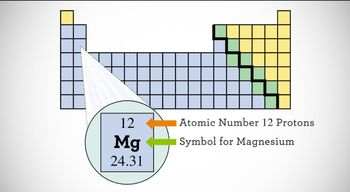09:35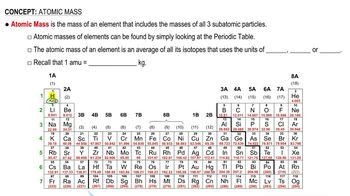02:19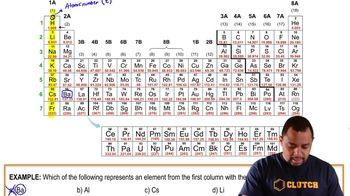02:06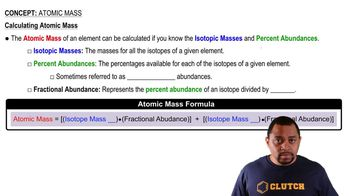02:00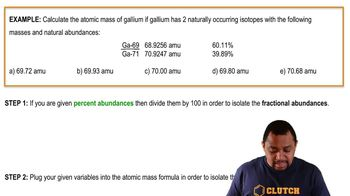02:03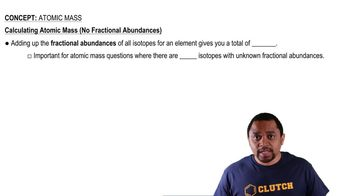00:40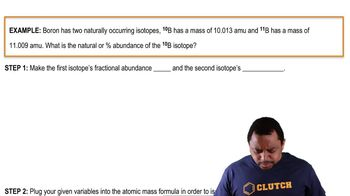03:38